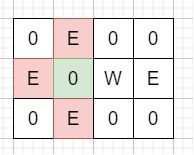# Bomb Enemy in C++

Suppose we have a 2D grid, here each cell is either a wall 'W', an enemy 'E' or that is empty '0', We have to find the maximum enemies we can kill using one bomb. The bomb kills all the enemies in the same row and column from the planted point until it hits the wall. And we can put bombs only on blank spaces.

So, if the input is likethen the output will be 3, as placing bomb at the green place, it will kill three enemies.

To solve this, we will follow these steps −

• ret := 0

• n := row count of grid, m := column count of grid

• Define an array colCnt of size m

• for initialize i := 0, when i < n, update (increase i by 1), do −

• for initialize j := 0, when j < m, update (increase j by 1), do −

• if j is zero or grid[i, j] is same as 'W', then −

• rowCnt := 0

• if grid[i, j] is same as 'W', then −

• k := j + 1

• Otherwise

• k := j

• for k < m and grid[i, k] is not equal to 'W', update (increase k by 1), do −

• rowCnt := rowCnt + 1 when (grid[i, k] is 'E'), otherwise 0

• if i is zero or grid[i, j] is same as 'W', then −

• colCnt[j] := 0

• if grid[i, j] is same as 'W', then −

• k := i + 1

• Otherwise

• k := i

• for k < n and grid[k, j] is not equal to 'W', update (increase k by 1), do −

• colCnt[j] := colCnt[j] + 1 when (grid[k, j] is 'E') otherwise 0

• if grid[i, j] is same as '0', then −

• ret := maximum of ret and rowCnt + colCnt[j]

• return ret

## Example

Let us see the following implementation to get better understanding −

Live Demo

#include <bits/stdc++.h>
using namespace std;
class Solution {
public:
int maxKilledEnemies(vector<vector<char>>& grid) {
int ret = 0;
int n = grid.size();
int m = n ? grid.size() : 0;
int rowCnt = 0;
vector<int< colCnt(m);
for (int i = 0; i < n; i++) {
for (int j = 0; j < m; j++) {
if (!j || grid[i][j] == 'W') {
rowCnt = 0;
int k;
if (grid[i][j] == 'W')
k = j + 1;
else
k = j;
for (; k < m && grid[i][k] != 'W'; k++) {
rowCnt += (grid[i][k] == 'E');
}
}
if (!i || grid[i][j] == 'W') {
colCnt[j] = 0;
int k;
if (grid[i][j] == 'W')
k = i + 1;
else
k = i;
for (; k < n && grid[k][j] != 'W'; k++) {
colCnt[j] += (grid[k][j] == 'E');
}
}
if (grid[i][j] == '0') {
ret = max(ret, rowCnt + colCnt[j]);
}
}
}
return ret;
}
};
main(){
Solution ob;
vector<vector<char>> v = {{'0','E','0','0'},{'E','0','W','E'},{'0','E','0','0'}};
cout << (ob.maxKilledEnemies(v));
}

## Input

{{'0','E','0','0'},{'E','0','W','E'},{'0','E','0','0'}}

## Output

3